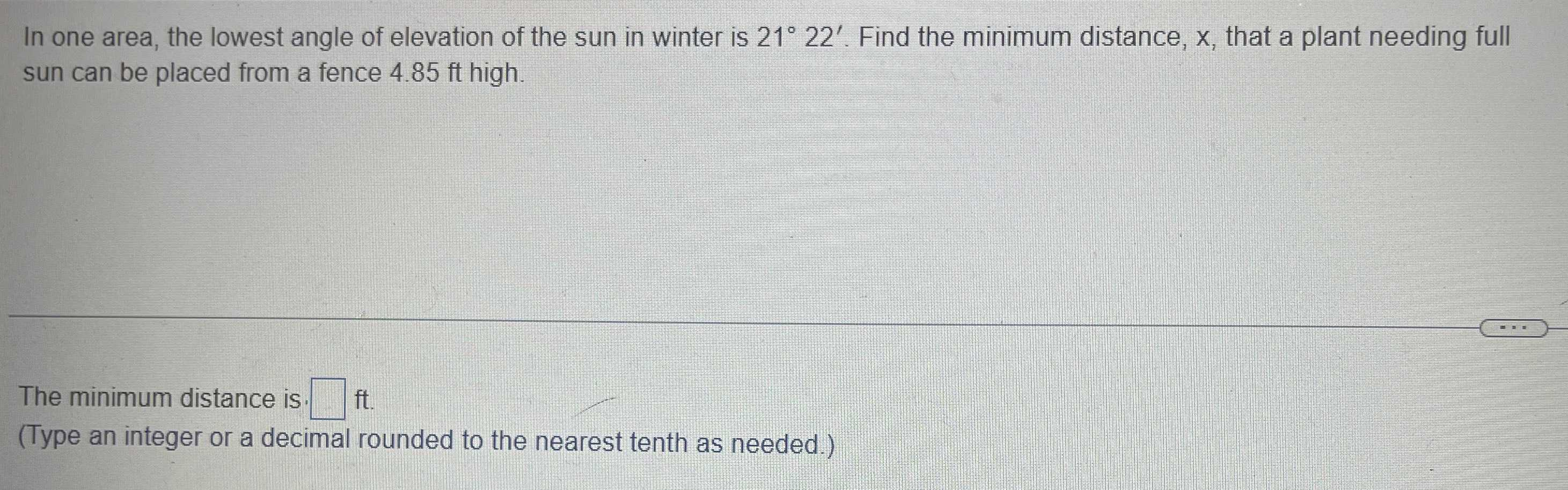### Still have math questions?

Trigonometry
QuestionIn one area, the lowest angle of elevation of the sun in winter is $$21 ^ { \circ } 22 ^ { \prime }$$ . Find the minimum distance, $$x$$ , that a plant needing full sun can be placed from a fence $$4.85 ft$$ high.

The minimum distance is. $$\square$$ ft.

(Type an integer or a decimal rounded to the nearest tenth as needed.)